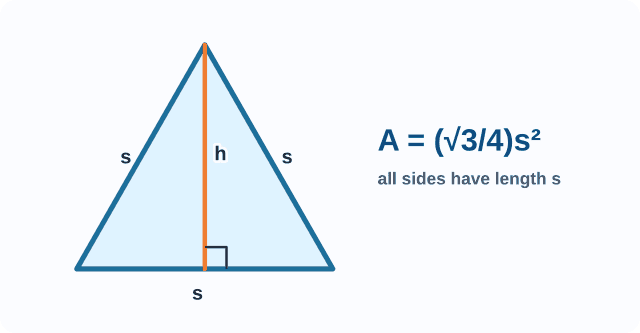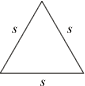# equiangular triangle

Equiangular Triangle

A triangle with three congruentangles.

Note: In Euclidean geometry, all equiangular triangles are equilateral and vice-versa. The angles of a Euclidean equiangular triangle each measure 60°.

 Equiangular (Equilateral) Triangles = length of a side